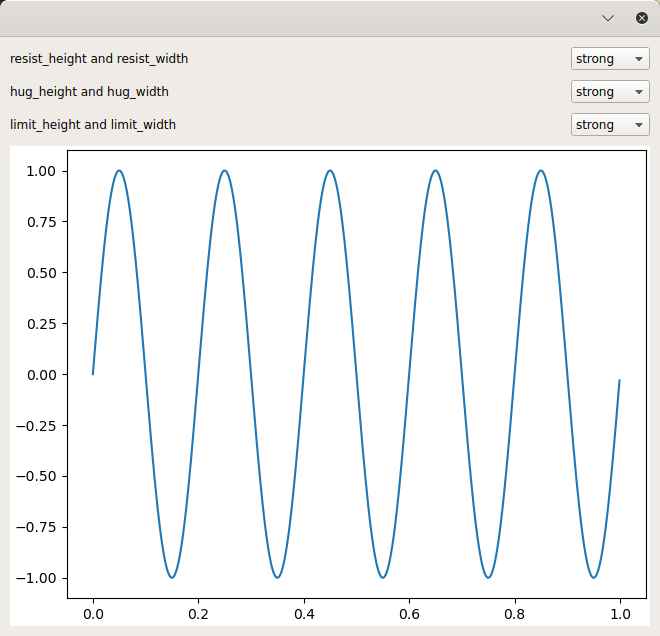# Mpl Canvas Size Example

An example of how to override tolkit-supplied size hints on your widget.

The toolkit-supplied size hint for MPLCanvas is 480 x 640 pixels, which prevents you from sizing the canvas smaller. This can be a problem when you are presenting a large grid of Matplotlib figures.

This example demonstrates how to override the tookit-supplied size hint for MPLCanvas. By setting resist_width, resist_height, hug_height, and hug_width to ‘ignore’, you can resize the figure almost freely in any direction.

This serves to demonstrate how the resist, hug and limit constraints interact to control the size of a widget.

resist:

If “strong”, the widget will not shrink smaller than the preferred size (i.e., the constraint width >= width_hint or height >= height_hint is set on the object).

hug:

If “strong”, the widget will not change from the preferred size (i.e., the constraint width == width_hint or height == height_hint is set on the object).

limit:

If “strong”, the widget will not expand larger than the preferred size (i.e., the constraint width <= width_hint and height <= height_hint is set on the object).

To allow a MPLCanvas to shrink smaller than the default size but not expand larger:

resist = ignore or weak hug = ignore or weak limit = strong

To allow a MPLCanvas to expand larger than the default size but not shrink smaller:

resist = strong hug = ignore or weak limit = ignore or weak

To disallow a MPLCanvas to change in size:

resist = strong, ignore or weak hug = strong limit = strong, ignore or weak

Tip

To see this example in action, download it from `mpl_canvas_size` and run:

```\$ enaml-run mpl_canvas_size.enaml
```

## Screenshot## Example Enaml Code

```#------------------------------------------------------------------------------
# Copyright (c) 2022, Nucleic Development Team.
#
#
# The full license is in the file LICENSE, distributed with this software.
#------------------------------------------------------------------------------
""" An example of how to override tolkit-supplied size hints on your widget.

The toolkit-supplied size hint for MPLCanvas is 480 x 640 pixels, which
prevents you from sizing the canvas smaller. This can be a problem when you are
presenting a large grid of Matplotlib figures.

This example demonstrates how to override the tookit-supplied size hint for
MPLCanvas. By setting resist_width, resist_height, hug_height, and hug_width to
'ignore', you can resize the figure almost freely in any direction.

This serves to demonstrate how the resist, hug and limit constraints interact
to control the size of a widget.

resist:
If "strong", the widget will not shrink smaller than the preferred size
(i.e., the constraint `width >= width_hint` or `height >= height_hint` is
set on the object).

hug:
If "strong", the widget will not change from the preferred size (i.e., the
constraint `width == width_hint` or `height == height_hint` is set on the
object).

limit:
If "strong", the widget will not expand larger than the preferred size
(i.e., the constraint `width <= width_hint` and `height <= height_hint` is
set on the object).

To allow a MPLCanvas to shrink smaller than the default size but not expand
larger:

resist = ignore or weak
hug = ignore or weak
limit = strong

To allow a MPLCanvas to expand larger than the default size but not shrink
smaller:

resist = strong
hug = ignore or weak
limit = ignore or weak

To disallow a MPLCanvas to change in size:

resist = strong, ignore or weak
hug = strong
limit = strong, ignore or weak

<< autodoc-me >>
"""

import matplotlib.pyplot as plt
import numpy as np

from enaml.widgets.api import (Form, Label, MPLCanvas, ObjectCombo, VGroup,
Window)

def make_figure():
# Constrained layout uses the kiwisolver engine and will ensure that the
# axes are formatted properly regardless of the size of the figure.
figure, axes = plt.subplots(constrained_layout=True)
x = np.arange(1000) / 1000
y = np.sin(2 * np.pi * 5 * x)
axes.plot(x, y)
return figure

enamldef Main(Window):

Form:
Label:
text = 'resist_height and resist_width'

ObjectCombo: resist:
items = ['strong', 'weak', 'ignore']

Label:
text = 'hug_height and hug_width'

ObjectCombo: hug:
items = ['strong', 'weak', 'ignore']

Label:
text = 'limit_height and limit_width'

ObjectCombo: limit:
items = ['strong', 'weak', 'ignore']

MPLCanvas: canvas:
resist_width << resist.selected
resist_height << resist.selected
hug_width << hug.selected
hug_height << hug.selected
limit_width << limit.selected
limit_height << limit.selected

# This specifies the minimum possible size for the window.
constraints = [(width >= 100) | 'strong', (height >= 100) | 'strong']
figure = make_figure()
```